# Executing file on Mac

Member Posts: 100Guru
edited November 2019 in Help
Has something changed with the execute programs operator?

```<?xml version="1.0" encoding="UTF-8"?><process version="9.4.001">
<context>
<input/>
<output/>
<macros/>
</context>
<operator activated="true" class="process" compatibility="9.4.001" expanded="true" name="Process">
<parameter key="logverbosity" value="init"/>
<parameter key="random_seed" value="2001"/>
<parameter key="send_mail" value="never"/>
<parameter key="process_duration_for_mail" value="30"/>
<parameter key="encoding" value="SYSTEM"/>
<process expanded="true">
<operator activated="true" class="text:create_document" compatibility="8.2.000" expanded="true" height="68" name="generate query" width="90" x="45" y="85">
<parameter key="text" value="aws athena start-query-execution --query-string &quot;select * from  table  where col0 like 'number';&quot; --result-configuration &quot;OutputLocation=s3://&quot;     &#10;"/>
<parameter key="label_type" value="nominal"/>
</operator>
<operator activated="true" class="text:write_document" compatibility="8.2.000" expanded="true" height="82" name="overwrite query" width="90" x="179" y="85">
<parameter key="file" value="/Users/robinmeisel/Dropbox/psyscore/master.sh"/>
<parameter key="overwrite" value="true"/>
<parameter key="encoding" value="SYSTEM"/>
</operator>
<operator activated="true" class="productivity:execute_program" compatibility="9.4.001" expanded="true" height="124" name="Execute Program" width="90" x="313" y="85">
<parameter key="command" value="chmod +x master.sh"/>
<parameter key="log_stdout" value="true"/>
<parameter key="log_stderr" value="false"/>
<parameter key="working_directory" value="/Users/execute"/>
<list key="env_variables"/>
</operator>
<operator activated="true" class="productivity:execute_program" compatibility="9.4.001" expanded="true" height="124" name="Execute Program (2)" width="90" x="447" y="85">
<parameter key="command" value="./master.sh"/>
<parameter key="log_stdout" value="true"/>
<parameter key="log_stderr" value="true"/>
<parameter key="working_directory" value="/Users/execute"/>
<list key="env_variables"/>
</operator>
<connect from_op="generate query" from_port="output" to_op="overwrite query" to_port="document"/>
<connect from_op="overwrite query" from_port="document" to_op="Execute Program" to_port="through 1"/>
<connect from_op="Execute Program" from_port="out" to_op="Execute Program (2)" to_port="through 1"/>
<portSpacing port="source_input 1" spacing="0"/>
<portSpacing port="sink_result 1" spacing="0"/>
</process>
</operator>
</process>```
I am now getting this error where the process used to work perfectly. Any ideas?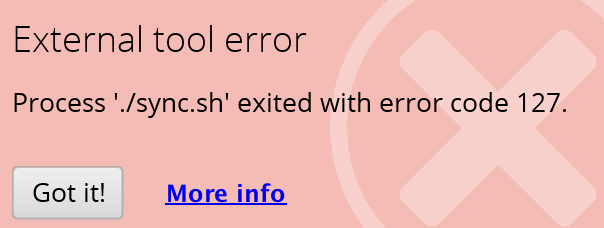Tagged:

• Member Posts: 100Guru
I am able to run the file from terminal, but not inside of the operator. This indicates that I have assigned the correct permissions to the file. The process works under older versions of RM where I have saved processes, but fails as soon as I update the process or run it under ver 9.4?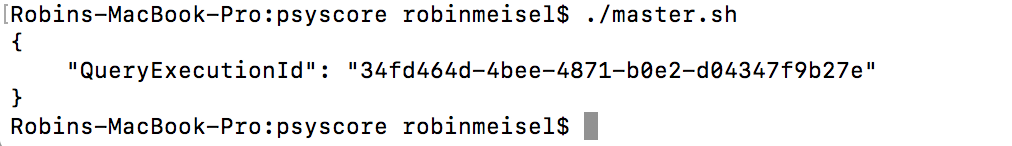• Team Lead Software Engineering Administrator, Moderator, Employee, Member, University Professor Posts: 1,873RM Engineering
Hi,

What is your OS X version?

Regards,
Marco
• Member Posts: 100Guru
10.13.6
• Member Posts: 100Guru
Does this have something to do with the fact that I am invoking the AWS CLI to perform Athena work? When I remove the was portion from the Create Document it works, but with the AWS it does not.
• Team Lead Software Engineering Administrator, Moderator, Employee, Member, University Professor Posts: 1,873RM Engineering
Hi,

Nothing has changed with the operator code itself in the past 4 years, so I would suspect it has to do something with an external change of some description.
According to https://stackoverflow.com/a/1763178/2333093, you get this if the command is not found or if +x is missing. I just noticed that your script is written to "/Users/robinmeisel/Dropbox/psyscore/master.sh", while your working directory is set to "/Users/execute". That explains why it complains that the file cannot be found.

I just tested it with an absolute path and a relative path + setting the working directory on (not yet officially supported) OS X 10.15, and it works fine.

Regards,
Marco
• 12Administrator, Moderator, Employee, RapidMiner Certified Analyst, Community Manager, Member, University Professor, PM Moderator Posts: 2,717Community Manager
edited November 2019
@robin I always run a chmod on the .sh file with Execute Program before doing another Execute Program with the same .sh file. I find the permissions get blocked (for reasons I cannot always figure out).
• Member Posts: 100Guru
edited November 2019
Hi Marco

Would be able to post the code that you have run, here I have set the paths to be the same but I am still getting an error?
```<?xml version="1.0" encoding="UTF-8"?><process version="9.4.001">
<context>
<input/>
<output/>
<macros/>
</context>
<operator activated="true" class="process" compatibility="9.4.001" expanded="true" name="Process">
<parameter key="logverbosity" value="init"/>
<parameter key="random_seed" value="2001"/>
<parameter key="send_mail" value="never"/>
<parameter key="process_duration_for_mail" value="30"/>
<parameter key="encoding" value="SYSTEM"/>
<process expanded="true">
<operator activated="false" class="set_macro" compatibility="9.4.001" expanded="true" height="68" name="Set Macro (3)" width="90" x="45" y="289">
<parameter key="macro" value="uuid"/>
<parameter key="value" value="25bb14c72b49dfbe594349d69790075e"/>
</operator>
<operator activated="true" class="utility:create_exampleset" compatibility="9.4.001" expanded="true" height="68" name="Create ExampleSet" width="90" x="179" y="34">
<parameter key="generator_type" value="attribute functions"/>
<parameter key="number_of_examples" value="1"/>
<parameter key="use_stepsize" value="false"/>
<list key="function_descriptions">
<parameter key="uuid" value="&quot;c95bc761eb81cfbba92046f7d8ec1b21&quot;"/>
</list>
<list key="numeric_series_configuration"/>
<list key="date_series_configuration"/>
<list key="date_series_configuration (interval)"/>
<parameter key="date_format" value="yyyy-MM-dd HH:mm:ss"/>
<parameter key="time_zone" value="SYSTEM"/>
<parameter key="column_separator" value=","/>
<parameter key="parse_all_as_nominal" value="false"/>
<parameter key="decimal_point_character" value="."/>
<parameter key="trim_attribute_names" value="true"/>
</operator>
<operator activated="true" class="loop_examples" compatibility="9.4.001" expanded="true" height="82" name="Loop Examples" width="90" x="447" y="34">
<parameter key="iteration_macro" value="eg_uuid"/>
<process expanded="true">
<operator activated="true" class="extract_macro" compatibility="9.4.001" expanded="true" height="68" name="Extract Macro (4)" width="90" x="179" y="34">
<parameter key="macro" value="uuid"/>
<parameter key="macro_type" value="data_value"/>
<parameter key="statistics" value="average"/>
<parameter key="attribute_name" value="uuid"/>
<parameter key="example_index" value="%{eg_uuid}"/>
</operator>
<operator activated="true" class="handle_exception" compatibility="9.4.001" expanded="true" height="68" name="Handle Exception (4)" width="90" x="313" y="34">
<process expanded="true">
<operator activated="true" class="concurrency:loop_files" compatibility="8.2.000" expanded="true" height="68" name="Loop Files (2)" width="90" x="112" y="136">
<parameter key="directory" value="/Users/robinmeisel/Dropbox/psyscore/sync"/>
<parameter key="filter_type" value="regex"/>
<parameter key="filter_by_regex" value=".*"/>
<parameter key="recursive" value="false"/>
<parameter key="enable_macros" value="true"/>
<parameter key="macro_for_file_name" value="del_file"/>
<parameter key="macro_for_file_type" value="file_type"/>
<parameter key="macro_for_folder_name" value="folder_name"/>
<parameter key="reuse_results" value="false"/>
<parameter key="enable_parallel_execution" value="true"/>
<process expanded="true">
<operator activated="true" class="delete_file" compatibility="9.4.001" expanded="true" height="68" name="Delete File" width="90" x="380" y="187">
<parameter key="file" value="/Users/robinmeisel/Dropbox/psyscore/sync/%{del_file}"/>
<parameter key="fail_if_missing" value="false"/>
</operator>
<portSpacing port="source_file object" spacing="0"/>
<portSpacing port="source_input 1" spacing="0"/>
<portSpacing port="sink_output 1" spacing="0"/>
</process>
<description align="center" color="transparent" colored="false" width="126">Delete from local drive</description>
</operator>
<portSpacing port="source_in 1" spacing="0"/>
<portSpacing port="sink_out 1" spacing="0"/>
</process>
<process expanded="true">
<portSpacing port="source_in 1" spacing="0"/>
<portSpacing port="sink_out 1" spacing="0"/>
</process>
</operator>
<operator activated="true" class="text:create_document" compatibility="8.2.000" expanded="true" height="68" name="generate query" width="90" x="313" y="238">
<parameter key="text" value="aws athena start-query-execution --query-string &quot;select * from  psyche.psychepsychemaster  where uuid like '%{uuid}' limit 1;&quot; --result-configuration &quot;OutputLocation=s3://psyscores/output/&quot;     "/>
<parameter key="label_type" value="nominal"/>
<description align="center" color="transparent" colored="false" width="126">Select master from S3</description>
</operator>
<operator activated="true" class="text:write_document" compatibility="8.2.000" expanded="true" height="82" name="overwrite query (4)" width="90" x="447" y="238">
<parameter key="file" value="/Users/robinmeisel/Dropbox/psyscore/master.sh"/>
<parameter key="overwrite" value="true"/>
<parameter key="encoding" value="SYSTEM"/>
</operator>
<operator activated="true" class="productivity:execute_program" compatibility="9.4.001" expanded="true" height="124" name="Execute Program (4)" width="90" x="581" y="238">
<parameter key="command" value="chmod +x master.sh"/>
<parameter key="log_stdout" value="true"/>
<parameter key="log_stderr" value="false"/>
<parameter key="working_directory" value="/Users/robinmeisel/Dropbox/psyscore"/>
<list key="env_variables"/>
</operator>
<operator activated="true" class="productivity:execute_program" compatibility="9.4.001" expanded="true" height="124" name="Execute Program (6)" width="90" x="715" y="238">
<parameter key="command" value="./master.sh"/>
<parameter key="log_stdout" value="true"/>
<parameter key="log_stderr" value="true"/>
<parameter key="working_directory" value="/Users/robinmeisel/Dropbox/psyscore"/>
<list key="env_variables"/>
</operator>
<operator activated="true" class="text:create_document" compatibility="8.2.000" expanded="true" height="68" name="generate query (3)" width="90" x="313" y="391">
<parameter key="text" value="aws athena start-query-execution --query-string &quot;select * from  psyche.psychepsycheemail  where uuid like '%{uuid}' limit 1;&quot; --result-configuration &quot;OutputLocation=s3://psyscores/output/&quot;     &#10;"/>
<parameter key="label_type" value="nominal"/>
<description align="center" color="transparent" colored="false" width="126">Select email from S3</description>
</operator>
<operator activated="true" class="text:write_document" compatibility="8.2.000" expanded="true" height="82" name="overwrite query (3)" width="90" x="447" y="391">
<parameter key="file" value="/Users/robinmeisel/Dropbox/psyscore/email.sh"/>
<parameter key="overwrite" value="true"/>
<parameter key="encoding" value="SYSTEM"/>
</operator>
<operator activated="true" class="productivity:execute_program" compatibility="9.4.001" expanded="true" height="124" name="Execute Program (5)" width="90" x="581" y="391">
<parameter key="command" value="chmod +x email.sh"/>
<parameter key="log_stdout" value="true"/>
<parameter key="log_stderr" value="true"/>
<parameter key="working_directory" value="/Users/robinmeisel/Dropbox/psyscore"/>
<list key="env_variables"/>
</operator>
<operator activated="true" class="productivity:execute_program" compatibility="9.4.001" expanded="true" height="124" name="Execute Program" width="90" x="715" y="391">
<parameter key="command" value="./email.sh"/>
<parameter key="log_stdout" value="true"/>
<parameter key="log_stderr" value="true"/>
<parameter key="working_directory" value="/Users/robinmeisel/Dropbox/psyscore"/>
<list key="env_variables"/>
</operator>
<operator activated="true" class="text:create_document" compatibility="8.2.000" expanded="true" height="68" name="generate queru (2)" width="90" x="313" y="544">
<parameter key="text" value="aws athena start-query-execution --query-string &quot;select * from  psyche.psychepsychemobile  where uuid like '%{uuid}' limit 1;&quot; --result-configuration &quot;OutputLocation=s3://psyscores/output/&quot;     &#10;"/>
<parameter key="label_type" value="nominal"/>
<description align="center" color="transparent" colored="false" width="126">Select mobile from S3</description>
</operator>
<operator activated="true" class="text:write_document" compatibility="8.2.000" expanded="true" height="82" name="overwrite query (2)" width="90" x="447" y="544">
<parameter key="file" value="/Users/robinmeisel/Dropbox/psyscore/mobile.sh"/>
<parameter key="overwrite" value="true"/>
<parameter key="encoding" value="SYSTEM"/>
</operator>
<operator activated="true" class="productivity:execute_program" compatibility="9.4.001" expanded="true" height="124" name="Execute Program (3)" width="90" x="581" y="544">
<parameter key="command" value="chmod +x mobile.sh"/>
<parameter key="log_stdout" value="true"/>
<parameter key="log_stderr" value="true"/>
<parameter key="working_directory" value="/Users/robinmeisel/Dropbox/psyscore"/>
<list key="env_variables"/>
</operator>
<operator activated="true" class="productivity:execute_program" compatibility="9.4.001" expanded="true" height="103" name="Execute Program (7)" width="90" x="715" y="544">
<parameter key="command" value="./mobile.sh"/>
<parameter key="log_stdout" value="true"/>
<parameter key="log_stderr" value="true"/>
<parameter key="working_directory" value="/Users/robinmeisel/Dropbox/psyscore"/>
<list key="env_variables"/>
</operator>
<operator activated="true" class="delay" compatibility="9.4.001" expanded="true" height="68" name="Delay" width="90" x="916" y="340">
<parameter key="delay" value="fixed"/>
<parameter key="delay_amount" value="20000"/>
<parameter key="min_delay_amount" value="0"/>
<parameter key="max_delay_amount" value="1000"/>
</operator>
<operator activated="true" class="concurrency:loop_files" compatibility="8.2.000" expanded="true" height="124" name="Loop Files" width="90" x="313" y="697">
<parameter key="directory" value="/Users/robinmeisel/Dropbox/psyscore/sync"/>
<parameter key="filter_type" value="regex"/>
<parameter key="filter_by_regex" value=".*.csv"/>
<parameter key="recursive" value="false"/>
<parameter key="enable_macros" value="true"/>
<parameter key="macro_for_file_name" value="file_extract"/>
<parameter key="macro_for_file_type" value="file_type_2"/>
<parameter key="macro_for_folder_name" value="folder_name_2"/>
<parameter key="reuse_results" value="false"/>
<parameter key="enable_parallel_execution" value="true"/>
<process expanded="true">
<operator activated="true" class="multiply" compatibility="9.4.001" expanded="true" height="124" name="Multiply" width="90" x="45" y="34"/>
<operator activated="true" class="handle_exception" compatibility="9.4.001" expanded="true" height="82" name="Handle Exception (3)" width="90" x="313" y="238">
<process expanded="true">
<operator activated="true" class="read_csv" compatibility="9.4.001" expanded="true" height="68" name="Read CSV (3)" width="90" x="112" y="187">
<parameter key="csv_file" value="/Users/robinmeisel/Dropbox/psyscore/sync/09c704d7-7bf4-4331-9800-2606edf6ee33.csv"/>
<parameter key="column_separators" value=","/>
<parameter key="trim_lines" value="false"/>
<parameter key="use_quotes" value="true"/>
<parameter key="quotes_character" value="&quot;"/>
<parameter key="escape_character" value="\"/>
<parameter key="comment_characters" value="#"/>
<parameter key="starting_row" value="1"/>
<parameter key="parse_numbers" value="true"/>
<parameter key="decimal_character" value="."/>
<parameter key="grouped_digits" value="false"/>
<parameter key="grouping_character" value=","/>
<parameter key="infinity_representation" value=""/>
<parameter key="date_format" value=""/>
<parameter key="first_row_as_names" value="true"/>
<list key="annotations"/>
<parameter key="time_zone" value="SYSTEM"/>
<parameter key="locale" value="English (United States)"/>
<parameter key="encoding" value="UTF-8"/>
<list key="data_set_meta_data_information">
<parameter key="0" value="uuid.true.polynominal.attribute"/>
<parameter key="1" value="id_number.true.polynominal.attribute"/>
<parameter key="2" value="title.true.polynominal.attribute"/>
<parameter key="3" value="firstname.true.polynominal.attribute"/>
<parameter key="4" value="lastname.true.polynominal.attribute"/>
<parameter key="5" value="gender.true.polynominal.attribute"/>
</list>
<parameter key="datamanagement" value="double_array"/>
<parameter key="data_management" value="auto"/>
</operator>
<operator activated="true" class="extract_macro" compatibility="9.4.001" expanded="true" height="68" name="Extract Macro (3)" width="90" x="380" y="187">
<parameter key="macro" value="uuid"/>
<parameter key="macro_type" value="data_value"/>
<parameter key="statistics" value="average"/>
<parameter key="attribute_name" value="uuid"/>
<parameter key="example_index" value="1"/>
<parameter key="firstname" value="firstname"/>
<parameter key="lastname" value="lastname"/>
<parameter key="id_number" value="id_number"/>
</list>
</operator>
<connect from_port="in 1" to_op="Read CSV (3)" to_port="file"/>
<connect from_op="Read CSV (3)" from_port="output" to_op="Extract Macro (3)" to_port="example set"/>
<connect from_op="Extract Macro (3)" from_port="example set" to_port="out 1"/>
<portSpacing port="source_in 1" spacing="0"/>
<portSpacing port="source_in 2" spacing="0"/>
<portSpacing port="sink_out 1" spacing="0"/>
<portSpacing port="sink_out 2" spacing="0"/>
</process>
<process expanded="true">
<portSpacing port="source_in 1" spacing="0"/>
<portSpacing port="source_in 2" spacing="0"/>
<portSpacing port="sink_out 1" spacing="0"/>
<portSpacing port="sink_out 2" spacing="0"/>
</process>
</operator>
<operator activated="true" class="handle_exception" compatibility="9.4.001" expanded="true" height="82" name="Handle Exception" width="90" x="313" y="136">
<process expanded="true">
<operator activated="true" class="read_csv" compatibility="9.4.001" expanded="true" height="68" name="Read CSV (2)" width="90" x="45" y="34">
<parameter key="csv_file" value="/Users/robinmeisel/Dropbox/psyscore/sync/1700898a-c9c8-408c-a2bf-6664e0c33703.csv"/>
<parameter key="column_separators" value=","/>
<parameter key="trim_lines" value="false"/>
<parameter key="use_quotes" value="true"/>
<parameter key="quotes_character" value="&quot;"/>
<parameter key="escape_character" value="\"/>
<parameter key="comment_characters" value="#"/>
<parameter key="starting_row" value="1"/>
<parameter key="parse_numbers" value="true"/>
<parameter key="decimal_character" value="."/>
<parameter key="grouped_digits" value="false"/>
<parameter key="grouping_character" value=","/>
<parameter key="infinity_representation" value=""/>
<parameter key="date_format" value="yyyy-MM-dd HH:mm:ss"/>
<parameter key="first_row_as_names" value="true"/>
<list key="annotations"/>
<parameter key="time_zone" value="SYSTEM"/>
<parameter key="locale" value="English (United States)"/>
<parameter key="encoding" value="UTF-8"/>
<list key="data_set_meta_data_information">
<parameter key="0" value="uuid.true.polynominal.attribute"/>
<parameter key="1" value="mobile.true.integer.attribute"/>
<parameter key="2" value="date.true.date_time.attribute"/>
</list>
<parameter key="datamanagement" value="double_array"/>
<parameter key="data_management" value="auto"/>
</operator>
<operator activated="true" class="select_attributes" compatibility="9.4.001" expanded="true" height="82" name="Select Attributes" width="90" x="179" y="34">
<parameter key="attribute_filter_type" value="subset"/>
<parameter key="attribute" value=""/>
<parameter key="attributes" value="mobile|uuid"/>
<parameter key="use_except_expression" value="false"/>
<parameter key="value_type" value="attribute_value"/>
<parameter key="use_value_type_exception" value="false"/>
<parameter key="except_value_type" value="time"/>
<parameter key="block_type" value="attribute_block"/>
<parameter key="use_block_type_exception" value="false"/>
<parameter key="except_block_type" value="value_matrix_row_start"/>
<parameter key="invert_selection" value="false"/>
<parameter key="include_special_attributes" value="false"/>
</operator>
<connect from_port="in 1" to_op="Read CSV (2)" to_port="file"/>
<connect from_op="Read CSV (2)" from_port="output" to_op="Select Attributes" to_port="example set input"/>
<connect from_op="Select Attributes" from_port="example set output" to_port="out 1"/>
<portSpacing port="source_in 1" spacing="0"/>
<portSpacing port="source_in 2" spacing="0"/>
<portSpacing port="sink_out 1" spacing="0"/>
<portSpacing port="sink_out 2" spacing="0"/>
</process>
<process expanded="true">
<portSpacing port="source_in 1" spacing="0"/>
<portSpacing port="source_in 2" spacing="0"/>
<portSpacing port="sink_out 1" spacing="0"/>
<portSpacing port="sink_out 2" spacing="0"/>
</process>
</operator>
<operator activated="true" class="handle_exception" compatibility="9.4.001" expanded="true" height="82" name="Handle Exception (2)" width="90" x="313" y="34">
<process expanded="true">
<parameter key="csv_file" value="/Users/robinmeisel/Dropbox/psyscore/sync/cf9b7ee4-3f08-4e99-9d6c-c794fa26417c.csv"/>
<parameter key="column_separators" value=","/>
<parameter key="trim_lines" value="false"/>
<parameter key="use_quotes" value="true"/>
<parameter key="quotes_character" value="&quot;"/>
<parameter key="escape_character" value="\"/>
<parameter key="comment_characters" value="#"/>
<parameter key="starting_row" value="1"/>
<parameter key="parse_numbers" value="true"/>
<parameter key="decimal_character" value="."/>
<parameter key="grouped_digits" value="false"/>
<parameter key="grouping_character" value=","/>
<parameter key="infinity_representation" value=""/>
<parameter key="date_format" value="yyyy-MM-dd HH:mm:ss"/>
<parameter key="first_row_as_names" value="true"/>
<list key="annotations"/>
<parameter key="time_zone" value="SYSTEM"/>
<parameter key="locale" value="English (United States)"/>
<parameter key="encoding" value="UTF-8"/>
<list key="data_set_meta_data_information">
<parameter key="0" value="uuid.true.polynominal.attribute"/>
<parameter key="1" value="email.true.polynominal.attribute"/>
<parameter key="2" value="date.true.date_time.attribute"/>
</list>
<parameter key="datamanagement" value="double_array"/>
<parameter key="data_management" value="auto"/>
</operator>
<operator activated="true" class="filter_examples" compatibility="9.4.001" expanded="true" height="103" name="Filter Examples" width="90" x="246" y="34">
<parameter key="parameter_expression" value=""/>
<parameter key="condition_class" value="custom_filters"/>
<parameter key="invert_filter" value="false"/>
<list key="filters_list">
<parameter key="filters_entry_key" value="email.contains.@/>
</list>
<parameter key=filters_logic_and" value="true"/>
</operator>
<connect from_port="in 1" to_op="Read CSV" to_port="file"/>
<connect from_op="Read CSV" from_port="output" to_op="Filter Examples" to_port="example set input"/>
<connect from_op="Filter Examples" from_port="example set output" to_port="out 1"/>
<portSpacing port="source_in 1" spacing="0"/>
<portSpacing port="source_in 2" spacing="0"/>
<portSpacing port="sink_out 1" spacing="0"/>
<portSpacing port="sink_out 2" spacing="0"/>
</process>
<process expanded="true">
<portSpacing port="source_in 1" spacing="0"/>
<portSpacing port="source_in 2" spacing="0"/>
<portSpacing port="sink_out 1" spacing="0"/>
<portSpacing port="sink_out 2" spacing="0"/>
</process>
</operator>
<connect from_port="file object" to_op="Multiply" to_port="input"/>
<connect from_op="Multiply" from_port="output 1" to_op="Handle Exception (2)" to_port="in 1"/>
<connect from_op="Multiply" from_port="output 2" to_op="Handle Exception" to_port="in 1"/>
<connect from_op="Multiply" from_port="output 3" to_op="Handle Exception (3)" to_port="in 1"/>
<connect from_op="Handle Exception (3)" from_port="out 1" to_port="output 2"/>
<connect from_op="Handle Exception" from_port="out 1" to_port="output 3"/>
<connect from_op="Handle Exception (2)" from_port="out 1" to_port="output 1"/>
<portSpacing port="source_file object" spacing="0"/>
<portSpacing port="source_input 1" spacing="0"/>
<portSpacing port="sink_output 1" spacing="0"/>
<portSpacing port="sink_output 2" spacing="0"/>
<portSpacing port="sink_output 3" spacing="0"/>
<portSpacing port="sink_output 4" spacing="0"/>
</process>
<description align="center" color="transparent" colored="false" width="126">Join CSV files from Athena</description>
</operator>
<operator activated="true" class="append" compatibility="9.4.001" expanded="true" height="82" name="Append (4)" width="90" x="581" y="697">
<parameter key="datamanagement" value="double_array"/>
<parameter key="data_management" value="auto"/>
<parameter key="merge_type" value="all"/>
</operator>
<operator activated="true" class="append" compatibility="9.4.001" expanded="true" height="82" name="Append (3)" width="90" x="581" y="901">
<parameter key="datamanagement" value="double_array"/>
<parameter key="data_management" value="auto"/>
<parameter key="merge_type" value="all"/>
</operator>
<operator activated="true" class="append" compatibility="9.4.001" expanded="true" height="82" name="Append" width="90" x="581" y="799">
<parameter key="datamanagement" value="double_array"/>
<parameter key="data_management" value="auto"/>
<parameter key="merge_type" value="all"/>
</operator>
<operator activated="true" class="concurrency:join" compatibility="9.4.001" expanded="true" height="82" name="Join" width="90" x="715" y="850">
<parameter key="remove_double_attributes" value="true"/>
<parameter key="join_type" value="left"/>
<parameter key="use_id_attribute_as_key" value="false"/>
<list key="key_attributes">
<parameter key="uuid" value="uuid"/>
</list>
<parameter key="keep_both_join_attributes" value="false"/>
</operator>
<operator activated="true" class="concurrency:join" compatibility="9.4.001" expanded="true" height="82" name="Join (2)" width="90" x="849" y="697">
<parameter key="remove_double_attributes" value="true"/>
<parameter key="join_type" value="inner"/>
<parameter key="use_id_attribute_as_key" value="false"/>
<list key="key_attributes">
<parameter key="uuid" value="uuid"/>
</list>
<parameter key="keep_both_join_attributes" value="false"/>
</operator>
<operator activated="true" class="extract_macro" compatibility="9.4.001" expanded="true" height="68" name="Extract Macro" width="90" x="983" y="697">
<parameter key="macro" value="uuid"/>
<parameter key="macro_type" value="data_value"/>
<parameter key="statistics" value="average"/>
<parameter key="attribute_name" value="uuid"/>
<parameter key="example_index" value="1"/>
<parameter key="email" value="email"/>
<parameter key="firstname" value="firstname"/>
<parameter key="lastname" value="lastname"/>
<parameter key="mobile" value="mobile"/>
<parameter key="id_number" value="id_number"/>
</list>
</operator>
<connect from_port="example set" to_op="Extract Macro (4)" to_port="example set"/>
<connect from_op="generate query" from_port="output" to_op="overwrite query (4)" to_port="document"/>
<connect from_op="overwrite query (4)" from_port="document" to_op="Execute Program (4)" to_port="through 1"/>
<connect from_op="Execute Program (4)" from_port="out" to_op="Execute Program (6)" to_port="through 1"/>
<connect from_op="generate query (3)" from_port="output" to_op="overwrite query (3)" to_port="document"/>
<connect from_op="overwrite query (3)" from_port="document" to_op="Execute Program (5)" to_port="through 1"/>
<connect from_op="Execute Program (5)" from_port="out" to_op="Execute Program" to_port="through 1"/>
<connect from_op="generate queru (2)" from_port="output" to_op="overwrite query (2)" to_port="document"/>
<connect from_op="overwrite query (2)" from_port="document" to_op="Execute Program (3)" to_port="through 1"/>
<connect from_op="Loop Files" from_port="output 1" to_op="Append" to_port="example set 1"/>
<connect from_op="Loop Files" from_port="output 2" to_op="Append (3)" to_port="example set 1"/>
<connect from_op="Loop Files" from_port="output 3" to_op="Append (4)" to_port="example set 1"/>
<connect from_op="Append (4)" from_port="merged set" to_op="Join (2)" to_port="left"/>
<connect from_op="Append (3)" from_port="merged set" to_op="Join" to_port="right"/>
<connect from_op="Append" from_port="merged set" to_op="Join" to_port="left"/>
<connect from_op="Join" from_port="join" to_op="Join (2)" to_port="right"/>
<connect from_op="Join (2)" from_port="join" to_op="Extract Macro" to_port="example set"/>
<portSpacing port="source_example set" spacing="0"/>
<portSpacing port="sink_example set" spacing="0"/>
<portSpacing port="sink_output 1" spacing="0"/>
</process>
</operator>
<connect from_op="Create ExampleSet" from_port="output" to_op="Loop Examples" to_port="example set"/>
<connect from_op="Loop Examples" from_port="example set" to_port="result 1"/>
<portSpacing port="source_input 1" spacing="0"/>
<portSpacing port="sink_result 1" spacing="0"/>
<portSpacing port="sink_result 2" spacing="0"/>
<description align="left" color="yellow" colored="false" height="105" resized="false" width="180" x="208" y="289">Snag list&lt;br&gt;1. Fix .sh execution&lt;br&gt;&lt;br&gt;</description>
</process>
</operator>
</process>

```

• Member Posts: 100Guru
edited November 2019
I have even tried running this with bulk shell file:

The code for the bulk file which I am able to execute from terminal is as follows
```./master.sh
./mobile.sh
./email.sh
./sync.sh
aws s3 rm s3://psyscores/output --recursive
```
I then attempt to execute this bulk file from RM.
```<?xml version="1.0" encoding="UTF-8"?><process version="9.4.001">
<context>
<input/>
<output/>
<macros/>
</context>
<operator activated="true" class="process" compatibility="9.4.001" expanded="true" name="Process">
<parameter key="logverbosity" value="init"/>
<parameter key="random_seed" value="2001"/>
<parameter key="send_mail" value="never"/>
<parameter key="process_duration_for_mail" value="30"/>
<parameter key="encoding" value="SYSTEM"/>
<process expanded="true">
<operator activated="true" class="productivity:execute_program" compatibility="9.4.001" expanded="true" height="103" name="Execute Program (2)" width="90" x="112" y="136">
<parameter key="command" value="chmod +x bulk.sh"/>
<parameter key="log_stdout" value="true"/>
<parameter key="log_stderr" value="true"/>
<parameter key="working_directory" value="/Users/robinmeisel/Dropbox/psyscore"/>
<list key="env_variables"/>
</operator>
<operator activated="true" class="productivity:execute_program" compatibility="9.4.001" expanded="true" height="103" name="Execute Program" width="90" x="246" y="136">
<parameter key="command" value="./bulk.sh"/>
<parameter key="log_stdout" value="true"/>
<parameter key="log_stderr" value="true"/>
<parameter key="working_directory" value="/Users/robinmeisel/Dropbox/psyscore"/>
<list key="env_variables"/>
</operator>
<portSpacing port="source_input 1" spacing="0"/>
<portSpacing port="sink_result 1" spacing="0"/>
</process>
</operator>
</process>```
This is the response I get (remembering that I was able to execute via terminal)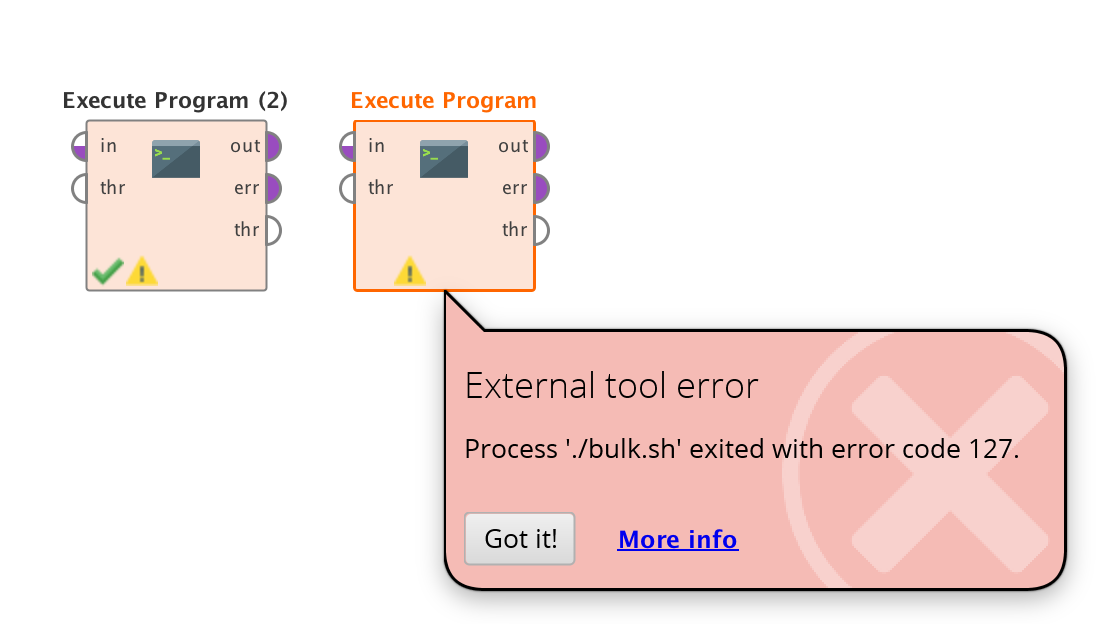• Team Lead Software Engineering Administrator, Moderator, Employee, Member, University Professor Posts: 1,873RM Engineering
Hi,

`touch /Users/mboeck/Downloads/test.txt`
I then ran chmod +x hello.sh, and afterwards ls -la, resulting in:
total 24
drwx------+  5 mboeck  staff   160  4 Nov 17:05 .
drwxr-xr-x+ 29 mboeck  staff   928  4 Nov 13:41 ..
[email protected]  1 mboeck  staff    38  4 Nov 13:42 hello.sh

My process for relative path:

```<?xml version="1.0" encoding="UTF-8"?><process version="9.5.000-BETA3">
<context>
<input/>
<output/>
<macros/>
</context>
<operator activated="true" class="process" compatibility="9.5.000-BETA3" expanded="true" name="Process">
<parameter key="logverbosity" value="init"/>
<parameter key="random_seed" value="2001"/>
<parameter key="send_mail" value="never"/>
<parameter key="process_duration_for_mail" value="30"/>
<parameter key="encoding" value="SYSTEM"/>
<process expanded="true">
<operator activated="true" class="retrieve" compatibility="9.5.000-BETA3" expanded="true" height="68" name="Retrieve Deals" width="90" x="45" y="34">
<parameter key="repository_entry" value="//Samples/data/Deals"/>
</operator>
<operator activated="true" class="productivity:execute_program" compatibility="9.5.000-BETA3" expanded="true" height="124" name="Execute Program" width="90" x="179" y="34">
<parameter key="command" value="./hello.sh"/>
<parameter key="log_stdout" value="true"/>
<parameter key="log_stderr" value="true"/>
<list key="env_variables"/>
</operator>
<connect from_op="Retrieve Deals" from_port="output" to_op="Execute Program" to_port="through 1"/>
<connect from_op="Execute Program" from_port="out" to_port="result 1"/>
<connect from_op="Execute Program" from_port="err" to_port="result 2"/>
<connect from_op="Execute Program" from_port="through 1" to_port="result 3"/>
<portSpacing port="source_input 1" spacing="0"/>
<portSpacing port="sink_result 1" spacing="0"/>
<portSpacing port="sink_result 2" spacing="0"/>
<portSpacing port="sink_result 3" spacing="0"/>
<portSpacing port="sink_result 4" spacing="0"/>
</process>
</operator>
</process>
```
My process with absolute path:
```<?xml version="1.0" encoding="UTF-8"?><process version="9.5.000-BETA3">
<context>
<input/>
<output/>
<macros/>
</context>
<operator activated="true" class="process" compatibility="9.5.000-BETA3" expanded="true" name="Process">
<parameter key="logverbosity" value="init"/>
<parameter key="random_seed" value="2001"/>
<parameter key="send_mail" value="never"/>
<parameter key="process_duration_for_mail" value="30"/>
<parameter key="encoding" value="SYSTEM"/>
<process expanded="true">
<operator activated="true" class="retrieve" compatibility="9.5.000-BETA3" expanded="true" height="68" name="Retrieve Deals" width="90" x="45" y="34">
<parameter key="repository_entry" value="//Samples/data/Deals"/>
</operator>
<operator activated="true" class="productivity:execute_program" compatibility="9.5.000-BETA3" expanded="true" height="124" name="Execute Program" width="90" x="179" y="34">
<parameter key="log_stdout" value="true"/>
<parameter key="log_stderr" value="true"/>
<list key="env_variables"/>
</operator>
<connect from_op="Retrieve Deals" from_port="output" to_op="Execute Program" to_port="through 1"/>
<connect from_op="Execute Program" from_port="out" to_port="result 1"/>
<connect from_op="Execute Program" from_port="err" to_port="result 2"/>
<connect from_op="Execute Program" from_port="through 1" to_port="result 3"/>
<portSpacing port="source_input 1" spacing="0"/>
<portSpacing port="sink_result 1" spacing="0"/>
<portSpacing port="sink_result 2" spacing="0"/>
<portSpacing port="sink_result 3" spacing="0"/>
<portSpacing port="sink_result 4" spacing="0"/>
</process>
</operator>
</process>
```

After both processes, I get the test.txt file inside the Downloads folder.

Regards,

Marco

• Member Posts: 100Guru
Thanks Marco. I have done extensive testing and I am able to execute multiple other .sh files via terminal and RM, the problem I have is that I am unable to execute those that call the amazon CLI, but am able to do manually via terminal. Each of the files below calls the AWS CLI and works via terminal but fails via RM.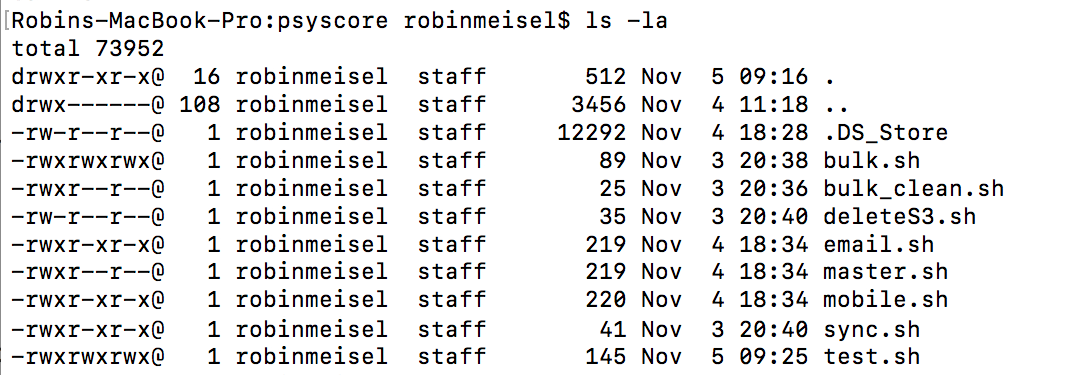What version of the CLI are you using? Maybe my issue lies there. Any other reason why I would not be able to call the CLI via shell?
• Team Lead Software Engineering Administrator, Moderator, Employee, Member, University Professor Posts: 1,873RM Engineering
Hi,

I was using whatever the standard Terminal on OS X 10.15.1 is.
The only explanation I have would be that for some reason the AWS CLI requires elevated permissions and when you start from the Terminal you have those, while Studio is started with normal permissions? I have no experience in permission management on OS X to be honest..

Regards,
Marco
• Member Posts: 100Guru
Have you installed the AWS CLI on your machine?

https://docs.aws.amazon.com/cli/latest/userguide/install-macos.html
• Team Lead Software Engineering Administrator, Moderator, Employee, Member, University Professor Posts: 1,873RM Engineering
Hi,

No, I did not, and as shown above, I only tested very simple scripts that had nothing to do with a 3rd party library. So it will have to do something with permissions. Did you maybe enable root access for your Terminal at some point previously?

Regards,
Marco
• Member Posts: 100Guru
Very difficult to answer, how would one enable root access for RapidMiner?

• 12Administrator, Moderator, Employee, RapidMiner Certified Analyst, Community Manager, Member, University Professor, PM Moderator Posts: 2,717Community Manager
• Member Posts: 100Guru
edited November 2019
Not the easiest way to test that the CLI is working, but yes I tried it and failed with the same error I am getting on my process.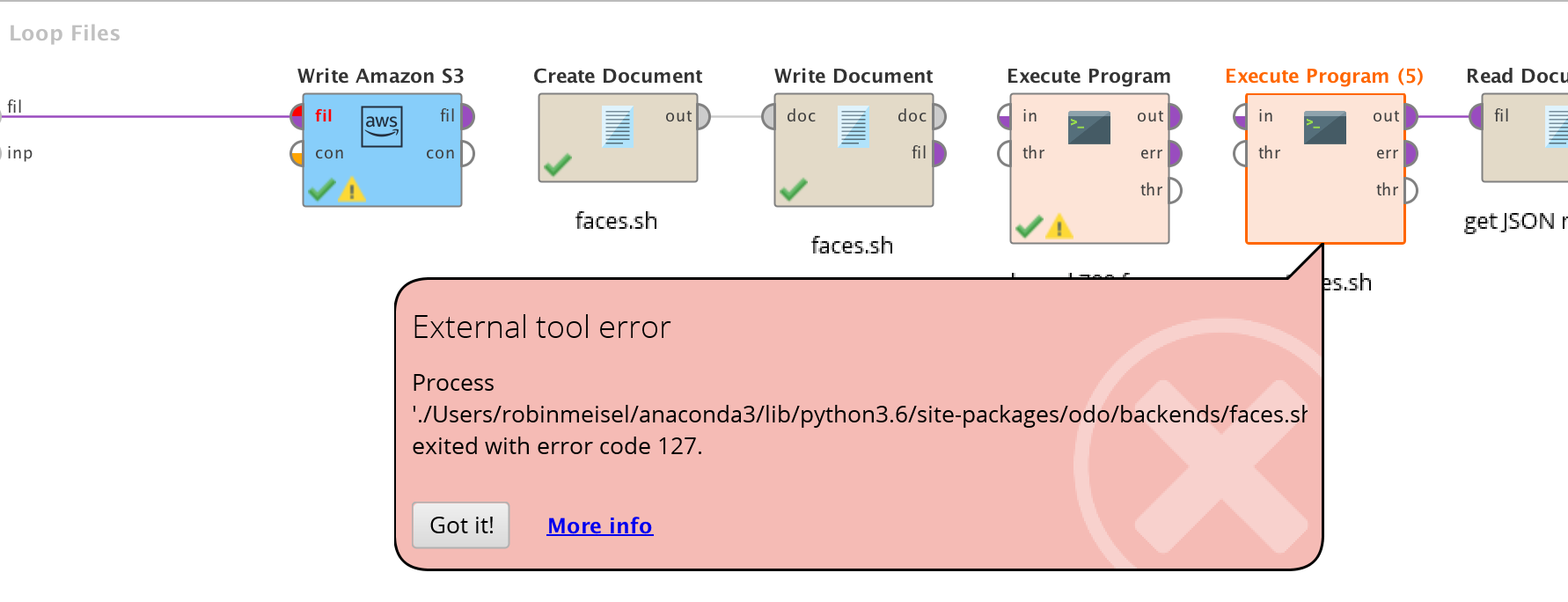Suggestions in terms of remediating? Reinstall the CLI? Reinstall RapidMiner?

PS: Can I update the Rekognition process to include remarks for people who will not be familiar with the CLI?
• 12Administrator, Moderator, Employee, RapidMiner Certified Analyst, Community Manager, Member, University Professor, PM Moderator Posts: 2,717Community Manager
@robin sorry I'm out of ideasI get that "error code 127" all the time when Execute Program. It almost always has to do with permissions in some way...but I am not the expert on this kind of thing.

As for better documentation on the Rekognition KB - yes please!